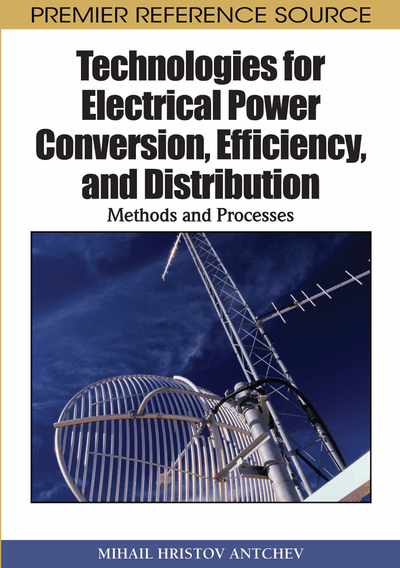# AC/DC Conversion

DOI: 10.4018/978-1-61520-647-6.ch004
Available
\$37.50
No Current Special Offers

## Abstract

Figure 1 displays a power electronic converter connected to the mains. In general, a power electronic converter is an electrical power converter – controlled or uncontrolled rectifier, AC regulator, compensator of reactive power, converter of phase number, active power filter. The converter supplies a load with power Pout, and in the same time it loads the mains with active power P and total power S.
Chapter Preview
Top

## Basic Indicators In Respect To The Supply Network

Figure 1 displays a power electronic converter connected to the mains. In general, a power electronic converter is an electrical power converter – controlled or uncontrolled rectifier, AC regulator, compensator of reactive power, converter of phase number, active power filter. The converter supplies a load with power Pout, and in the same time it loads the mains with active power P and total power S.

Power factor is defined as a ratio of active power P to total apparent power S:

(4.1)

If the voltage and current of the supply network are with non-sinusoidal waveform, they contain DC component and they can be presented in Fourier series, then the active power is given as:

(4.2) where in Uk and Ik are the effective values of the kth harmonic of the voltage and current, respectively, andis the displacement angle.

Total power is a product of the effective values of the source voltage U and the source current I:

(4.3) where inand(4.4)

After substituting (4.4) in (4.1), it is found:

(4.5)

The mains voltage is usually accepted to be of a pure sinusoidal waveform and it does not contain a DC component, and the source current is usually accepted to be of non-sinusoidal waveform. So, it is derived:

(4.6)

From (4.6) it is seen, that the power factor is a product of two variables, the highest value of each of them can be equal to 1. The two variables are:

Besides the distortion factor, the current non-sinusoidal waveform is also characterized by harmonic distortion factor or a total harmonic distortion defined as:

(4.7)

It is easy to find the relationship between the two coefficients:

(4.8) or

(4.9)

## Complete Chapter List

Search this Book:
Reset